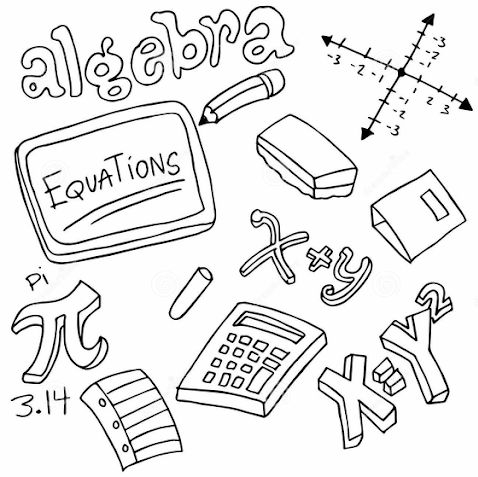# Change Of Subject Formulae

Welcome to Class !!

In today’s Mathematics class, We will be discussing Change of Subject of Formula. We hope you enjoy the class!##### CHANGE OF SUBJECT OF FORMULA

A formula is a general equation involving two or more unknowns. An example is the formula a = ¶r2  which gives the area of the circle in terms of its radius r. In this formula, a is called the subject of the formula.

Simplifying a formula by substitution

Example:

1. Given mx+c=y, express x in terms of m, c, and y. Find the value of x if y=10, c=2 and m is 4

Solution:

1)  mx+c= y,    mx=y-c

To find the value of X, when  y= 10 , m=4and c=2

X=

Evaluation: If I=  PRT

• Make p the subject and find the value of p if I = 10, R= 4, and T= 5.

#### CHANGING THE SUBJECT OF A FORMULA

When a variable which forms a part of the formula is made subject, we say we have changed the subject of the formula.

Examples:

1. In the formula S=2¶r(r+h), make h the subject.
2. Make m the subject if

Solution:

1) S=2¶r(r+h)

S=2¶r 2 +2¶rh

S- 2¶r 2 =2¶rh

S-2¶r2 =h

2¶r

2)

Cross multiply:  FT =mv-mu

FT =m(v-u)

EVALUATION

1. Make r the subject if V =¶r 2h
2. Make u the subject if 1/f =1/v + 1/u

1. Exam focus for J.S.C.E Pg. 212 -216
2. Essential Maths for J.S.S.3 Pg. 47-54

WEEKEND ASSIGNMENT

1. Express a in terms of u, v, and t in v= u+at
• (a)  a = vu-t
•  (b) a= v-u
•  (c) a =  $\frac{v+u}{t}$

1.   If Z=2p +3, find the value of Z when p=1. (a) Z=2, (b) Z= 5, (c) Z =7
2. In the relation X = $\frac{m–6y}{6}$  , how would you write m in terms of x and y?

(a) m=x-6   (b) m = 6x -6y (c) m =6x +6y

1. Express n in terms of s, a, and L if

1. Make U the subject if V2 = U2  +2as.

(a) U= V 2 -2as   (b) U = (V 2 -2as)    (c)       V2  – 2a

THEORY

1. Given L = arn-1, make a the subject of the formula.
2. If S + $\frac{2t}{d}$, make t the subject of the formula. Find the value of t if S=1, d= 2.

We have come to the end of this class. We do hope you enjoyed the class?

Should you have any further question, feel free to ask in the comment section below and trust us to respond as soon as possible.

In our next class, we will be talking about Measure of Central Tendency. We are very much eager to meet you there.

Are you a Parent? Share your quick opinion and win free 2-month Premium Subscription

### 2 thoughts on “Change Of Subject Formulae”

1.V=u+2as

2.johnchibuike2468

Please i am a student, and i don’t understand the 2 last examples in changing the subject of a formula. Please can you deeply explain for us.

Don`t copy text!